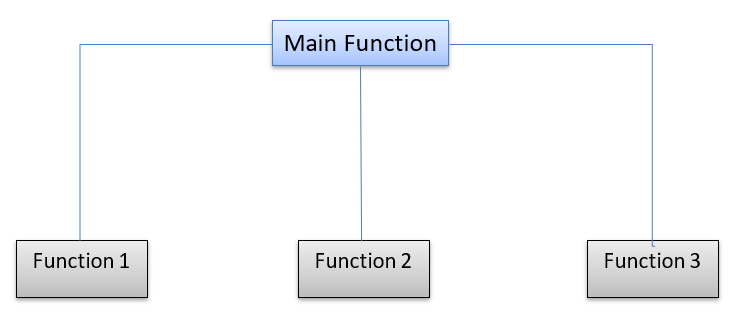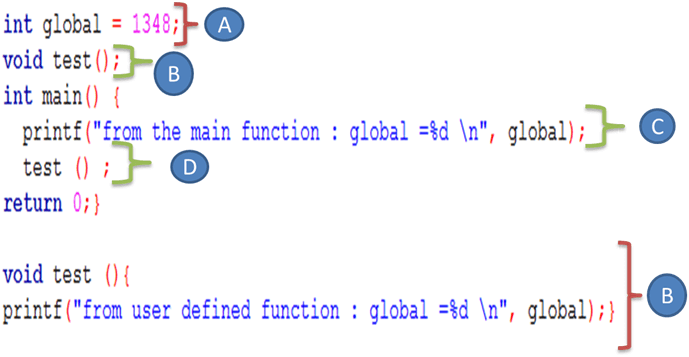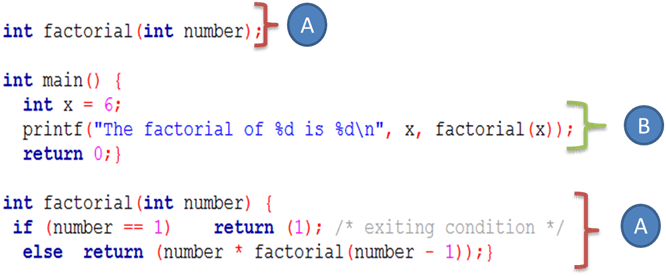# Functions in C Programming with Examples: Recursive, Inline

## What is a Function?

A function in programming is a reusable block of code that makes a program easier to test, understand and can be modified easily without changing the calling program. Functions to divide the code and modularize the program for better and effective results. In short, a larger program is divided into various subprograms which are called as functions.When you divide a large program into various functions, it becomes easy to manage each function individually. Whenever an error occurs in the program, you can easily investigate faulty functions and correct only those errors. You can easily call and use functions whenever they are required which automatically leads in saving time and space.

In this tutorial, you will learn-

## Library Vs. User-defined Functions

Every ‘C’ program has at least one function which is the main function, but a program can have any number of functions. The main () function is a starting point of a program.

In ‘C’ programming, functions are divided into two types:

1. Library functions
2. User-defined functions

The difference between the library and user-defined functions is that we do not need to write a code for a library function. It is already present inside the header file which we always include at the beginning of a program. You just have to type the name of a function and use it along with the proper syntax. Printf, scanf are the examples of a library function.

Whereas, a user-defined function is a type of function in which we have to write a body of a function and call the function whenever we require the function to perform some operation in our program.

A user-defined function is always written by the user, but later it can be a part of ‘C’ library. It is a major advantage of ‘C’ programming.

In ‘C’ programming functions are divided into three activities such as,

1. Function declaration
2. Function definition
3. Function call

## Function Declaration

Function declaration means writing a name of a program. It is a compulsory part for using functions in code. In a function declaration, we just specify the name of a function that we are going to use in our program like a variable declaration. We cannot use a function unless it is declared in a program. A function declaration is also called “Function prototype.”

The function declarations (called prototype) are usually done above the main () function and take the general form:

```return_data_type function_name (data_type arguments);
```
• The return_data_type: is the data type of the value function returned back to the calling statement.
• The function_name: is followed by parentheses
• Arguments names with their data type declarations optionally are placed inside the parentheses.

We consider the following program that shows how to declare a cube function to calculate the cube value of an integer variable

```#include <stdio.h>
/*Function declaration*/
/*End of Function declaration*/
int main() {
```

Keep in mind that a function does not necessarily return a value. In this case, the keyword void is used.

For example, the output_message function declaration indicates that the function does not return a value: void output_message();

## Function Definition

Function definition means just writing the body of a function. A body of a function consists of statements which are going to perform a specific task. A function body consists of a single or a block of statements. It is also a mandatory part of a function.

```int add(int a,int b)	//function body
{
int c;
c=a+b;
return c;
}
```

## Function call

A function call means calling a function whenever it is required in a program. Whenever we call a function, it performs an operation for which it was designed. A function call is an optional part in a program.

```  result = add(4,5);
```

Here, is th complete code:

```#include <stdio.h>
int add(int a, int b);	//function declaration
int main()
{
int a=10,b=20;
int c=add(10,20); 	//function call
getch();
}
int add(int a,int b)	//function body
{
int c;
c=a+b;
return c;
}
```

Output:

```Addition:30
```

## Function Arguments

A function’s arguments are used to receive the necessary values by the function call. They are matched by position; the first argument is passed to the first parameter, the second to the second parameter and so on.

By default, the arguments are passed by value in which a copy of data is given to the called function. The actually passed variable will not change.

We consider the following program which demonstrates parameters passed by value:

```int add (int x, int y);
int main() {
int a, b, result;
a = 5;
b = 10;
result = add(a, b);
printf("%d + %d\ = %d\n", a, b, result);
return 0;}
int add (int x, int y) {
x += y;
return(x);}
```

The program output is:

`5 + 10 = 15 `

Keep in mind that the values of a and b were passed to add function were not changed because only its value was passed into the parameter x.

## Variable Scope

Variable scope means the visibility of variables within a code of the program.

In C, variables which are declared inside a function are local to that block of code and cannot be referred to outside the function. However, variables which are declared outside all functions are global and accessible from the entire program. Constants declared with a #define at the top of a program are accessible from the entire program. We consider the following program which prints the value of the global variable from both main and user defined function :

```#include <stdio.h>
int global = 1348;
void test();
int main() {
printf("from the main function : global =%d \n", global);
test () ;
return 0;}

void test (){
printf("from user defined function : global =%d \n", global);}
```

Result:

```
from the main function : global =1348
from user defined function : global =1348
```

We discuss the program details:1. We declare an integer globale variable with 1348 as initial value.
2. We declare and define a test() function which neither takes arguments nor returns a value. This function only prints the globale variable value to demonstrate that the global variables can be accessed anywhere in the program.
3. We print the global variable within the main function.
4. We call the test function in orde to print the global variable value.

In C, when arguments are passed to function parameters, the parameters act as local variables which will be destroyed when exiting the function.

When you use global variables, use them with caution because can lead to errors and they can change anywhere in a program. They should be initialized before using.

## Static Variables

The static variables have a local scope. However, they are not destroyed when exiting the function. Therefore, a static variable retains its value forever and can be accessed when the function is re-entered. A static variable is initialized when declared and needs the prefix static.

The following program uses a static variable:

```#include <stdio.h>
void say_hi();
int main() {
int i;
for (i = 0; i < 5; i++) { say_hi();}
return 0;}
void say_hi() {
static int calls_number = 1;
printf("Hi number %d\n", calls_number);
calls_number ++; } ```

The program displays :

```Hi number 1
Hi number 2
Hi number 3
Hi number 4
Hi number 5
```

## Recursive Functions

Consider the factorial of a number which is calculated as follow 6! =6* 5 * 4 * 3 * 2 * 1.

This calculation is done as repeatedly calculating fact * (fact -1) until fact equals 1.

A recursive function is a function which calls itself and includes an exit condition in order to finish the recursive calls. In the case of the factorial number calculation, the exit condition is fact equals to 1. Recursion works by “stacking” calls until the exiting condition is true.

For example:

```#include <stdio.h>
int factorial(int number);
int main() {
int x = 6;
printf("The factorial of %d is %d\n", x, factorial(x));
return 0;}
int factorial(int number) {
if (number == 1)    return (1); /* exiting condition */
else
return (number * factorial(number - 1));
} ```

The program displays:

` The factorial of 6 is 720 `

Here,we discuss program details:1. We declare our recursive factorial function which takes an integer parameter and returns the factorial of this parameter.This function will call itself and decrease the number until the exiting, or the base condition is reached. When the condition is true, the previously generated values will be multiplied by each other, and the final factorial value is returned.
2. We declare and initialize an integer variable with value”6″ and then print its factorial value by calling our factorial function.

Consider the following chart to more understand the recursive mechanism which consists of calling the function its self until the base case or stopping condition is reached, and after that, we collect the previous values:## Inline Functions

Functions are used to store the most frequently used instructions. It is used for modularizing the program.

Whenever a function is called, the instruction pointer jumps to the function definition. After executing a function, instruction pointer falls back to the statement from where it jumped to the function definition.

Whenever we use functions, we require an extra pointer head to jump to the function definition and return to the statement. To eliminate the need of such pointer heads, we use inline functions.

In an inline function, a function call is directly replaced by an actual program code. It does not jump to any block because all the operations are performed inside the inline function.

Inline functions are mostly used for small computations. They are not suitable when large computing is involved.

An inline function is similar to the normal function except that keyword inline is place before the function name. Inline functions are created with the following syntax:

```inline function_name ()
{
//function definition
}
```

Let us write a program to implement an inline function.

```inline int add(int a, int b)		//inline function declaration
{
return(a+b);
}
int main()
{
getch();
}
```

Output:

```Addition: 30
```

Above program demonstrates the use of an inline function for addition of two numbers. As we can see, we have returned the addition on two numbers within the inline function only without writing any extra lines. During function call we have just passed values on which we have to perform addition.

## Summary

• A function is a mini-program or a subprogram.
• Functions are used to modularize the program.
• Library and user-defined are two types of functions.
• A function consists of a declaration, function body, and a function call part.
• Function declaration and body are mandatory.
• A function call can be optional in a program.
• C program has at least one function; it is the main function ().
• Each function has a name, data type of return value or a void, parameters.
• Each function must be defined and declared in your C program.
• Keep in mind that ordinary variables in a C function are destroyed as soon as we exit the function call.
• The arguments passed to a function will not be changed because they passed by value none by address.
• The variable scope is referred to as the visibility of variables within a program
• There are global and local variables in C programming

.